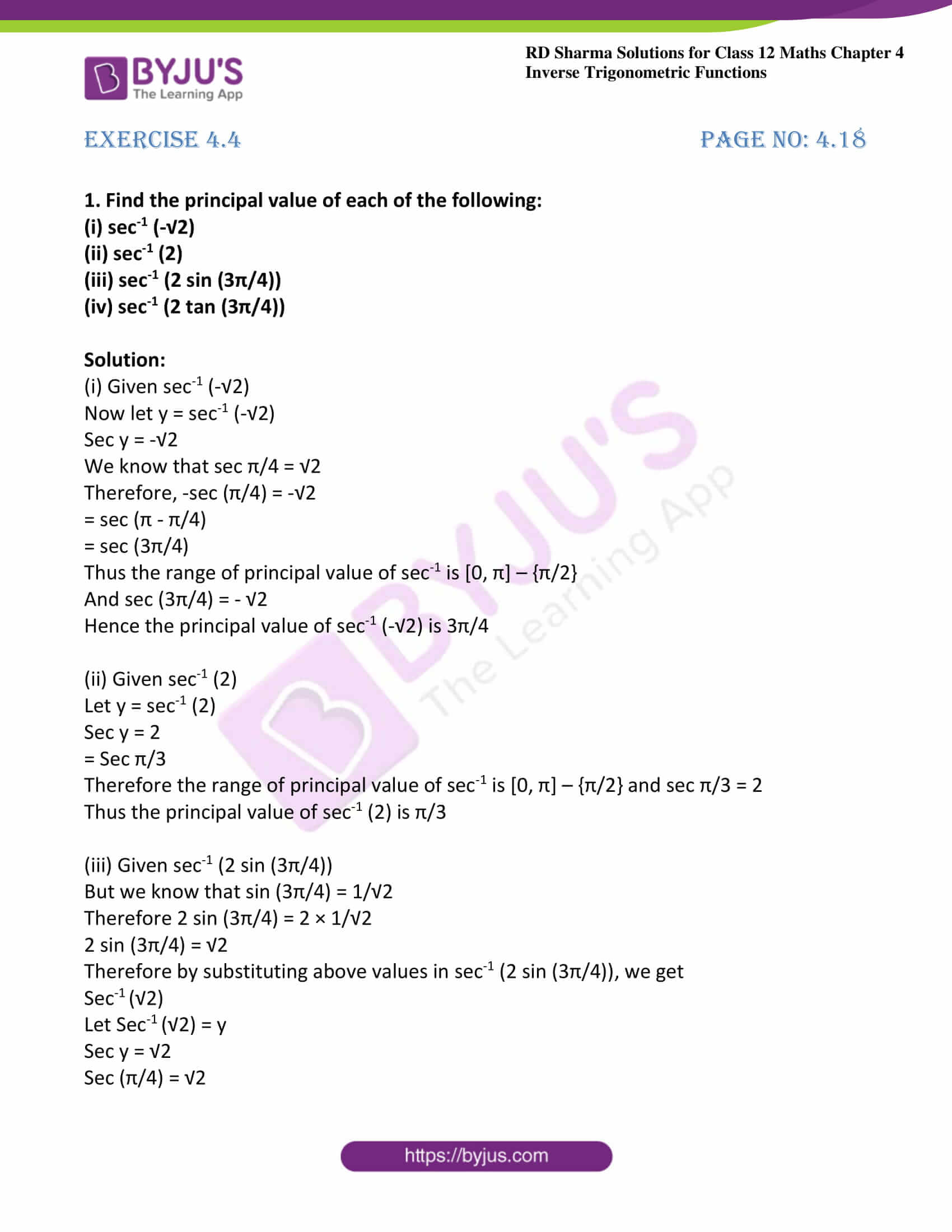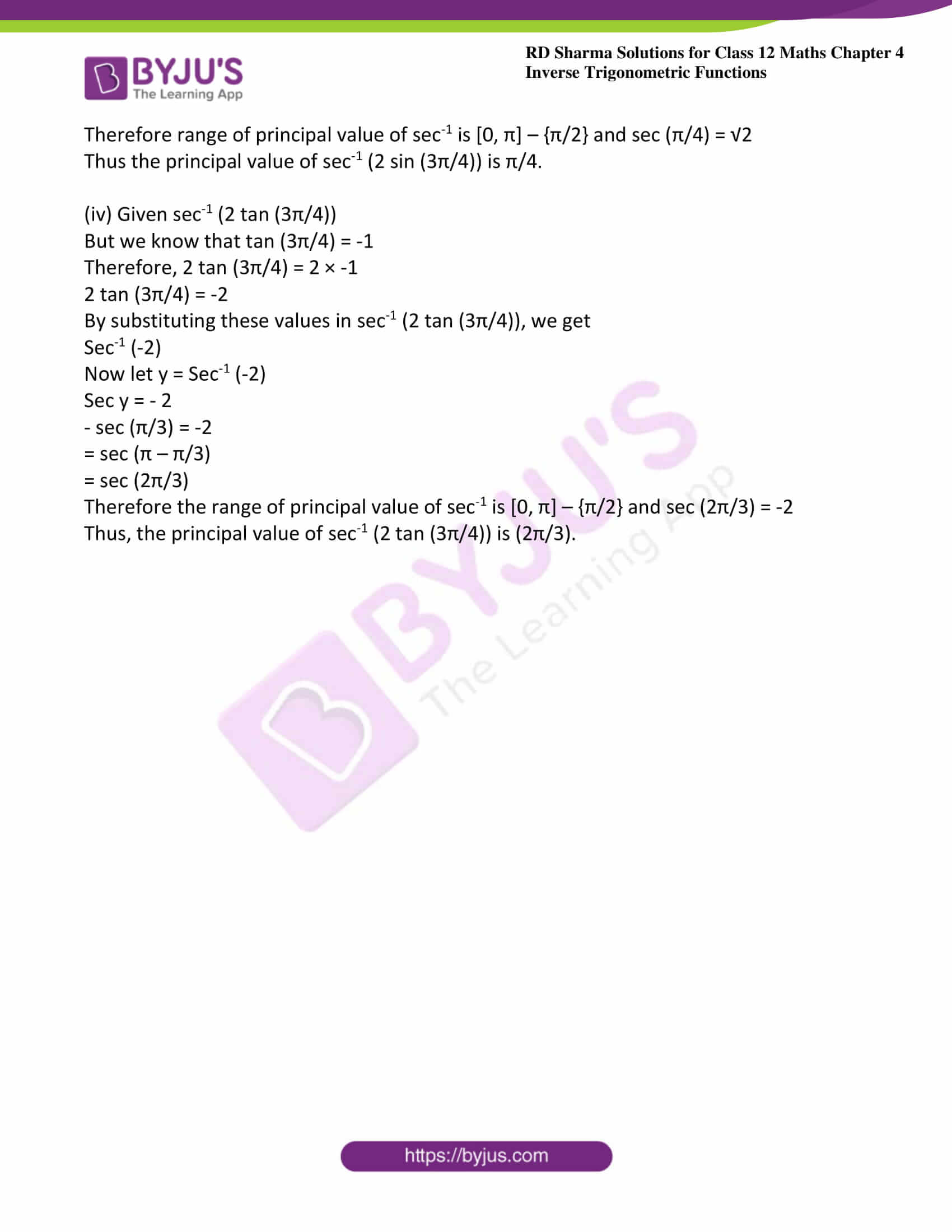# RD Sharma Solutions For Class 12 Maths Exercise 4.4 Chapter 4 Inverse Trigonometric Functions

RD Sharma Solutions Class 12 Maths Exercise 4.4 Chapter 4 Inverse Trigonometric Functions is provided here. This exercise explains inverse of secant function and range lies in secant function. These concepts are explained in simple language by a set of expert faculty at BYJU’S. The problems are solved in an interactive manner to make it interesting for the students while solving them.

The primary aim of preparing RD Sharma Solutions is to help students with their exam preparation. To score well in the exam, students can refer to RD Sharma Solutions Class 12 Maths Chapter 4 Inverse Trigonometric Functions Exercise 4.4 PDF, which are provided below.

## Download the PDF of RD Sharma Solutions For Class 12 Chapter 4 – Inverse Trigonometric Functions Exercise 4.4### Exercise 4.4 Page No: 4.18

1. Find the principal value of each of the following:

(i) sec-1 (-√2)

(ii) sec-1 (2)

(iii) sec-1 (2 sin (3π/4))

(iv) sec-1 (2 tan (3π/4))

Solution:

(i) Given sec-1 (-√2)

Now let y = sec-1 (-√2)

Sec y = -√2

We know that sec π/4 = √2

Therefore, -sec (π/4) = -√2

= sec (π – π/4)

= sec (3π/4)

Thus the range of principal value of sec-1 is [0, π] – {π/2}

And sec (3π/4) = – √2

Hence the principal value of sec-1 (-√2) is 3π/4

(ii) Given sec-1 (2)

Let y = sec-1 (2)

Sec y = 2

= Sec π/3

Therefore the range of principal value of sec-1 is [0, π] – {π/2} and sec π/3 = 2

Thus the principal value of sec-1 (2) is π/3

(iii) Given sec-1 (2 sin (3π/4))

But we know that sin (3π/4) = 1/√2

Therefore 2 sin (3π/4) = 2 × 1/√2

2 sin (3π/4) = √2

Therefore by substituting above values in sec-1 (2 sin (3π/4)), we get

Sec-1 (√2)

Let Sec-1 (√2) = y

Sec y = √2

Sec (π/4) = √2

Therefore range of principal value of sec-1 is [0, π] – {π/2} and sec (π/4) = √2

Thus the principal value of sec-1 (2 sin (3π/4)) is π/4.

(iv) Given sec-1 (2 tan (3π/4))

But we know that tan (3π/4) = -1

Therefore, 2 tan (3π/4) = 2 × -1

2 tan (3π/4) = -2

By substituting these values in sec-1 (2 tan (3π/4)), we get

Sec-1 (-2)

Now let y = Sec-1 (-2)

Sec y = – 2

– sec (π/3) = -2

= sec (π – π/3)

= sec (2π/3)

Therefore the range of principal value of sec-1 is [0, π] – {π/2} and sec (2π/3) = -2

Thus, the principal value of sec-1 (2 tan (3π/4)) is (2π/3).

### Access other exercises of RD Sharma Solutions For Class 12 Chapter 4 – Inverse Trigonometric Functions

Exercise 4.1 Solutions

Exercise 4.2 Solutions

Exercise 4.3 Solutions

Exercise 4.5 Solutions

Exercise 4.6 Solutions

Exercise 4.7 Solutions

Exercise 4.8 Solutions

Exercise 4.9 Solutions

Exercise 4.10 Solutions

Exercise 4.11 Solutions

Exercise 4.12 Solutions

Exercise 4.13 Solutions

Exercise 4.14 Solutions